Created July 13, 2018

# Water Purifier Helper

Through this project, we are trying to make water quality checker and help people who are negatively affected by polluted water purifier.

161## Things used in this project

### Hardware components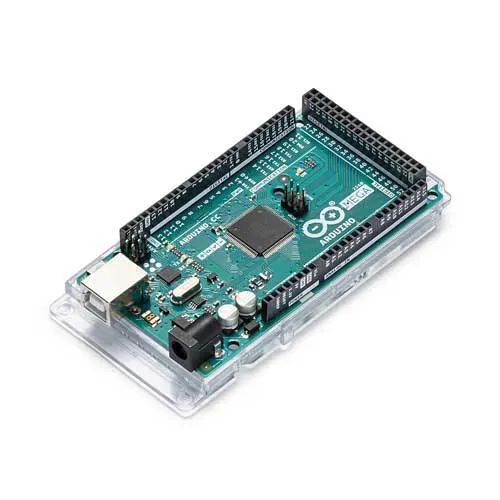Arduino Mega 2560
×1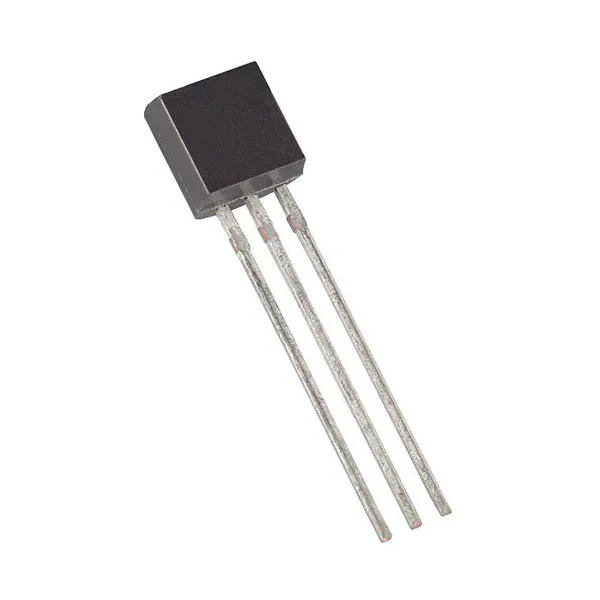Maxim Integrated DS18B20 Programmable Resolution 1-Wire Digital Thermometer
×1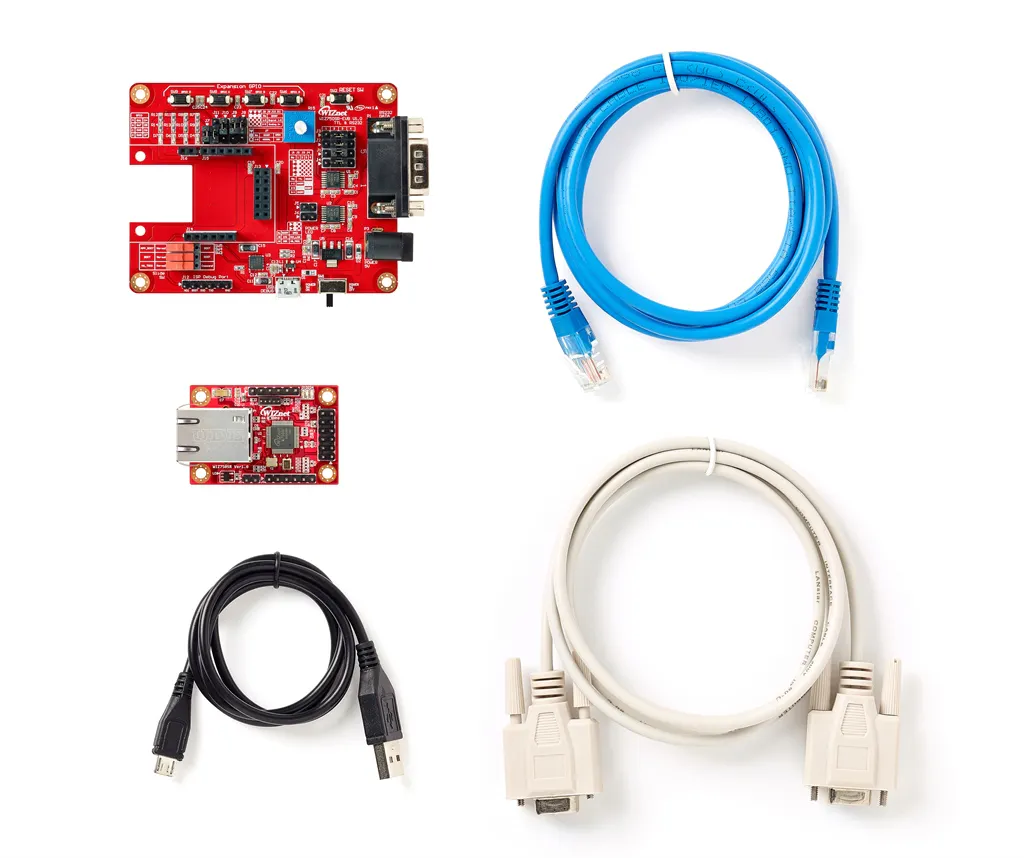WIZnet WIZ750SR-TTL-EVB Kit
×1
 DFRobot sen0161
×1
 DFRobot sen0189
×1

### Software apps and online servicesArduino IDEMQTT

Read more

## Schematics

### Circuit Flow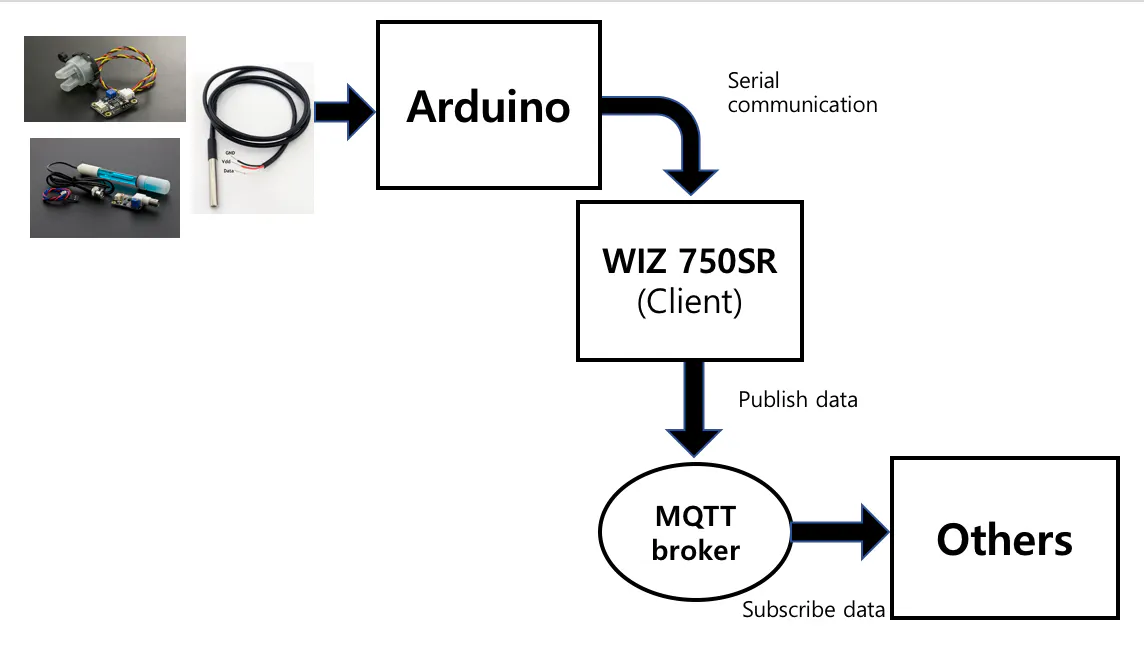### Arduino Circuit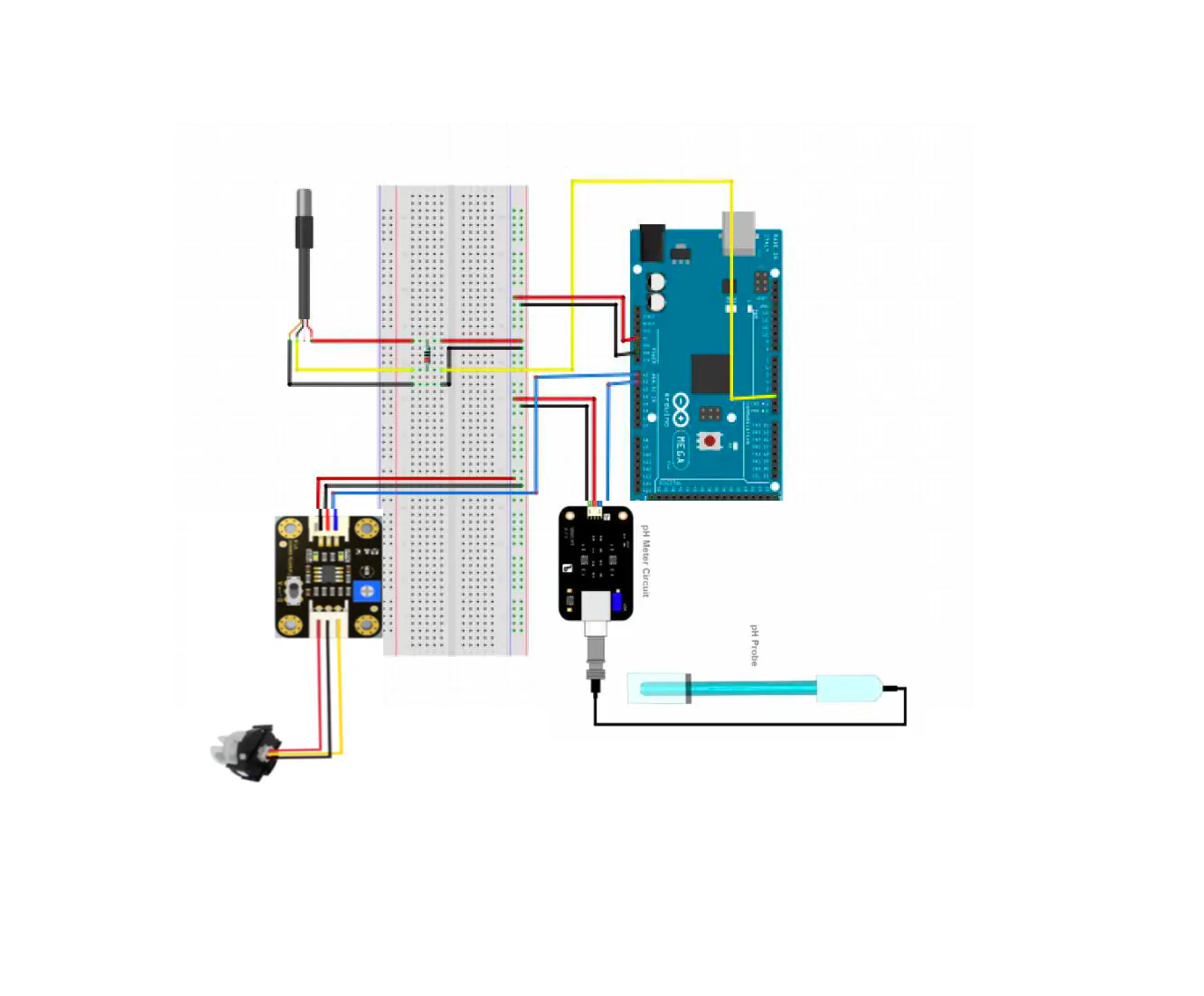### Appinventor blocks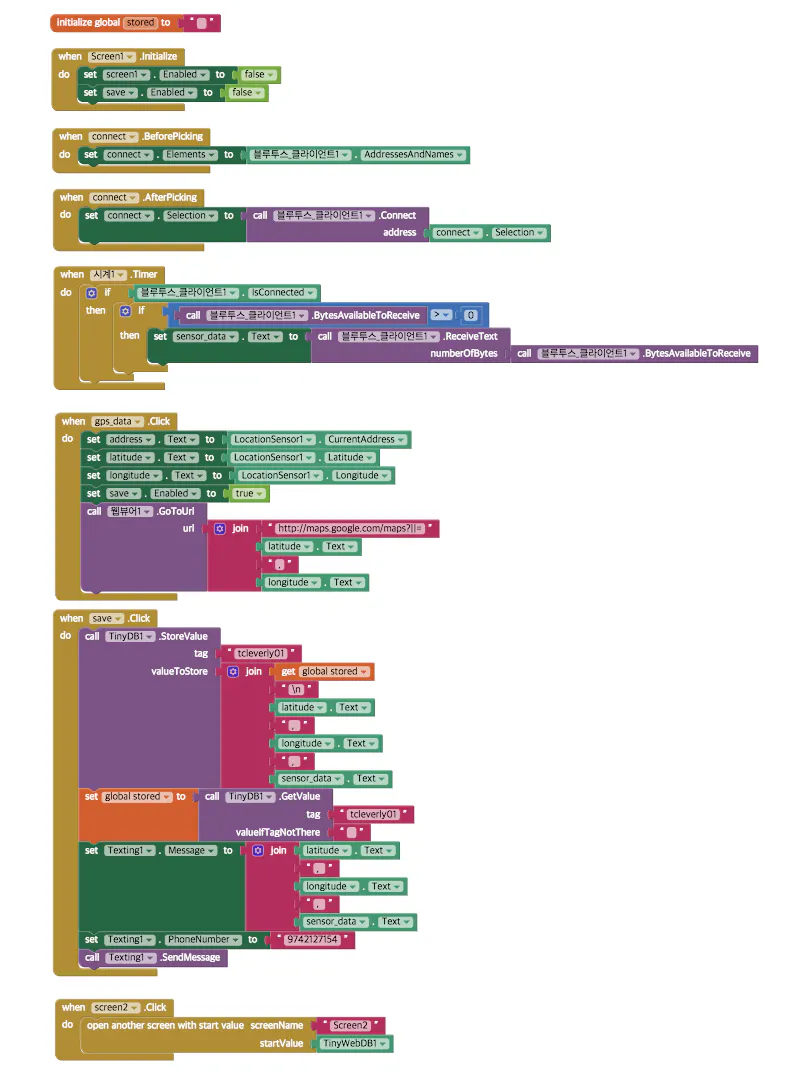## Code

### Arduino code

Arduino
```#include <SoftwareSerial.h>
#include <OneWire.h>
#include <DallasTemperature.h>
#define SensorPin A1            //pH meter Analog output to Arduino Analog Input 0
#define Offset 0.00            //deviation compensate
#define samplingInterval 20
#define printInterval 800
#define ArrayLenth  40    //times of collection
#define ONE_WIRE_BUS 2 //온도센서 디지털 핀번호

OneWire oneWire(ONE_WIRE_BUS);
DallasTemperature sensors(&oneWire);

int pHArray[ArrayLenth];   //Store the average value of the sensor feedback
int pHArrayIndex = 0;
int pin = 2;

//int DS18S20 = 3;
//OneWire ds(DS18S20);
SoftwareSerial myserial(10,11);//TX,RX
void setup() {
// put your setup code here, to run once:
Serial.begin(9600);
myserial.begin(9600);
sensors.begin();
}

void loop() {
// put your main code here, to run repeatedly:
static unsigned long samplingTime = millis();
static unsigned long printTime = millis();
static float pHValue, voltage, tak;
//sensors.requestTemperatures();

//Serial.println(takSensor);
//Serial.println(temp);

if (millis() - samplingTime > samplingInterval)
{
pHArray[pHArrayIndex++] = analogRead(SensorPin);

if (pHArrayIndex == ArrayLenth)
{
pHArrayIndex = 0;
}
voltage = avergearray(pHArray, ArrayLenth) * 5.0 / 1024;
pHValue = 3.5 * voltage + Offset;
samplingTime = millis();
}

if ((millis() - printTime > printInterval))
{
int sensorValue = analogRead(A0);
float volt = sensorValue * (5.0 / 1024.0);

//Serial.print(sensors.getTempCByIndex(0));
sensors.requestTemperatures();
float c = sensors.getTempCByIndex(0);

if(pHValue>5.8 && pHValue < 8.4 && volt >= 3.8 && volt <= 4.4)
{
myserial.print("Quality is Good, \n");
myserial.print("Temp : ");
myserial.print(c);
myserial.println(" C");

Serial.print("Good, ");
Serial.print("Temp : ");
Serial.println(c);
}
else
{
myserial.print("Quality is Bad, \n");
myserial.print("Temp : ");
myserial.print(c);
myserial.println(" C");

Serial.print("Bad, ");
Serial.print("Temp : ");
Serial.println(c);
}
delay(3000); //3초마다 Refresh
}
}

double avergearray(int* arr, int number) {
int i;
int max, min;
double avg;
long amount = 0;
if (number <= 0) {
Serial.println("Error number for the array to avraging!/n");
return 0;
}
if (number < 5) { //less than 5, calculated directly statistics
for (i = 0; i < number; i++) {
amount += arr[i];
}
avg = amount / number;
return avg;
} else {
if (arr < arr) {
min = arr; max = arr;
}
else {
min = arr; max = arr;
}
for (i = 2; i < number; i++) {
if (arr[i] < min) {
amount += min;      //arr<min
min = arr[i];
} else {
if (arr[i] > max) {
amount += max;  //arr>max
max = arr[i];
} else {
amount += arr[i]; //min<=arr<=max
}
}//if
}//for
avg = (double)amount / (number - 2);
}//if
return avg;
}
```

### Mbed

C/C++
```#include "mbed.h"
#include "SPI.h"
#include "MQTTEthernet.h"
#include "MQTTClient.h"

#define ECHO_SERVER_PORT   7

Serial pc(USBTX, USBRX);   //Enabling the Serial transmission between WIZ750SR and PC.
Serial serial(D1,D0);      // Enabling Serial transmission betwenn Wiz750SR and W7500.
char c="";

int main(void) {
printf("Wait a second...\r\n");
char* topic = "Vending machine";
MQTTEthernet ipstack = MQTTEthernet();

MQTT::Client<MQTTEthernet, Countdown> client = MQTT::Client<MQTTEthernet, Countdown>(ipstack);

char* hostname = "172.16.73.4";   //Give the IP Address of the MQTT Broker.
int port = 1883;                  // Port number of the MQTT broker.

int rc = ipstack.connect(hostname, port);

if (rc != 0)
printf("rc from TCP connect is %d\n", rc);
printf("Topic: %s\r\n",topic);

MQTTPacket_connectData data = MQTTPacket_connectData_initializer;
data.MQTTVersion = 3;
data.clientID.cstring = "parents";

if ((rc = client.connect(data)) == 0)
printf("rc from MQTT connect is %d\n", rc);

while (true) {
int i;
c[i]=0;
if (serial.readable()) // if anything available/ readable in Serial port
{

for(i=0;i<=13;i++){
char c1 =serial.getc();
c[i] = c1;
}
pc.printf("The value returned is %s ",c);
MQTT::Message message;
char buf;
sprintf(buf, "%s", c1);
message.qos = MQTT::QOS0;
message.retained = false;
message.dup = false;

message.payload = (void*)c;
message.payloadlen = strlen(c);

rc = client.publish("IOT", message);
pc.printf("Rc result: %c \n ",rc);
client.yield(60);
}
}
}
```

## Credits

### 이민석

1 project • 0 followers

### 백지원

0 projects • 0 followers

### 이중원

0 projects • 0 followers

### Huijin Yang

0 projects • 0 followers